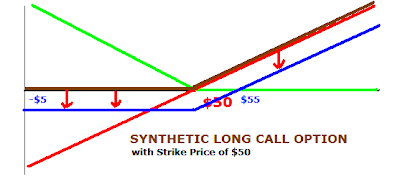Synthetic Long Call Option Trading: Explained with Example: Options, Futures, Derivatives & Commodity Trading

# Synthetic Long Call Option Trading: Explained with Example

This series of articles will be dedicated to explaining Synthetic Long Call Option Trading.

The term "Synthetic" comes from the reason that this position is constructed as an artificial option position - it resembles a real call option, but since it is constructed with a combination, that's why it is known as a "Synthetic Call Option".

What is the Synthetic Long Call Option Trading Position?
If we combine a position in actual stock and a long put with proper strike price and lot sizes (as explained below), then we get a Synthetic Long Call Option position.

How can one construct the Synthetic Long Call Option Trading Position?
The Synthetic long call option position can be constructed simply by taking a long position in stock and adding a long put position to it.
Care should be taken about the numbers of shares to be bought and the lot size of the long put options to be bought. Also strike price should be selected accordingly.

- Buy X no. of shares of the underlying at price \$Y
- Buy X no. of Long put options on the same underlying, with a strike price of \$Y

As explained in the below example, the price of undelrying stock was \$50 (Y) and suppose we purchase 100 shares (X). Hence, we should purchase 100 Put options with ATM strike price of \$50.
(Want to know what is ITM, OTM, ATM in Options? See Moneyness of Options - OTM, ATM, ITM Options)

Here is a small demo:
You purchase 100 shares of Microsoft stock at a buy price of \$50 per share and you take the following position:The benefit is that as long as the stock price remains above \$50, you are in profit.
But, the problem with this position is that as long as the stock price remains below your BUY price of \$50, then you are in loss. Hence, as a trader, you wish to limit your losses or even better, make your losses to zero. That will be possible only when the above position of Long stock can be transformed into a "Long Call" position, which has a theoretical flat or zero loss (without price being considered). (see Long Call Option Trading Explained)
So how can that be achieved?
We only need to cut down or nullify the loss area, and that area is below \$50. Hence, let's add such a position which takes this loss making portion to zero. And the way to nullify something is to add equal and opposite position to it - i.e. something which has equal value but opposite direction [since x + (-x) = 0]. (To understand option position additions see the details example in Addition of Option positions Explained)
So if we look below \$50 portion of the aboe long stock position function, we observe that the payoff function is NEGATIVE (as it is below the horizontal ZERO line) and it is INCREASING (because as we move from left to right, the payoff function increases in value).
So to nullify this, we need to add something which is POSITIvE (i.e. above the ZERO line) and DECREASING (as we move from left to right, the graph should come down).

Please note that our focus is only on nullifying the loss region (below the \$50) and keep the profit making position as it is. Hence, we dont make any additions to the loss making position. Or simply put, adding a ZERO to X will not change X (since X + 0 =X), so in the profit region, we add a zero, and in the loss position we add an equal and opposite value position.
So this is how this position to be added will look:Now, let's add both these positions together and what we get is follows:
Between \$0 and \$50, the RED and GREEN colored functions will cancel each other as they are opposite in directions but equal in magnitude. Above \$50, the LONG STOCK position (RED) and Zero GREEN option position will give you net RED position. Overall, we get net BROWN position which is a combination of LONG STOCK position (RED) and LONG PUT position (GREEN).
Now by adding these 2 postions, what we get is a net payoff function (BROWN color) which looks similar to a LONG CALL option position. But since it is made by a combination, it is known as a SYNTHETIC LONG CALL option position.How did we achieve this? We had a long position in stock (RED), we added something which is represented by the GREEN payoff function and got a BROWN colored payoff function similar to a call option position. But what is the GREEN payoff function like? It is actually a LONG PUT OPTION. (See Long Put Option Trading Explained

Hence, by adding a long put to a long stock position we managed to synthetically or artificially create a synthetic long call position.
But please note that the long put will not come for free, it will cost some money. Assume that it costs you \$5, so let's shift the entire BROWN payoff function down by \$5 and what we get is a BLUE colored payoff function, which reflects the true and realistic payoff function of a the Synthetic Long call position with all the prices taken into consideration.
One question that should come to your mind - Why should someone create a Synthetic Long Call Option position when you already have the "real" call options available for trading? Let's see the answer in Synthetic Long Call Option Trading: Profit & Loss Calculations
 Posted by Shobhit See All Articles with